Courses

# Test: Chemical Equilibrium- 2

## 25 Questions MCQ Test Physical Chemistry | Test: Chemical Equilibrium- 2

Description
This mock test of Test: Chemical Equilibrium- 2 for Chemistry helps you for every Chemistry entrance exam. This contains 25 Multiple Choice Questions for Chemistry Test: Chemical Equilibrium- 2 (mcq) to study with solutions a complete question bank. The solved questions answers in this Test: Chemical Equilibrium- 2 quiz give you a good mix of easy questions and tough questions. Chemistry students definitely take this Test: Chemical Equilibrium- 2 exercise for a better result in the exam. You can find other Test: Chemical Equilibrium- 2 extra questions, long questions & short questions for Chemistry on EduRev as well by searching above.
QUESTION: 1

Solution:
QUESTION: 2

Solution:
QUESTION: 3

### Does Le-Chatelier’s principle predict a change of equilibrium concentrations for the following reaction if the gas mixture is compressed: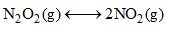Solution:
QUESTION: 4

The plot given below shows the relation between (1/T) and log(s), where S is the solubility of an electrolyte AB and T is the temperature in Kelvin. What conclusion can be drawn from the plot: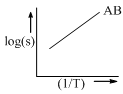Solution:
QUESTION: 5

A mixture of 2 mole each of helium and an unknown gas (normal boiling point = 0°C) is kept in a 22.4 L flask. If the flask is cooled to 0.1°C, the resultant pressure (in atm) inside the flask is:

Solution:
QUESTION: 6

For the complete oxidation of 100 g of Cyclohexanol to cyclohexanon, the quantity of CrO3 required is (assuming 100% chemical yield) [Atomic wt. of Cr = 52)

Solution:
QUESTION: 7

For the reaction: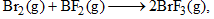the equilibrium constant at 2000 K and 1.0 bar is 5.25. When the pressure is increased by 8-fold, the equilibrium constant:

Solution:
QUESTION: 8

In a chemical reaction,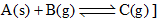The total pressure at equilibrium is 6 atm. The value of equilibrium constant is:

Solution:
QUESTION: 9

If the equilibrium constants for the reactions 1 and 2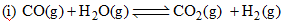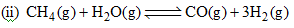Are K1 and K2, the equilibrium constant for the reaction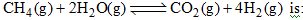Solution:
QUESTION: 10

Consider the reaction,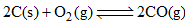at equilibrium,

The equilibrium can be shifted towards the forward direction by:

Solution:
QUESTION: 11

When a reversible reaction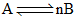is followed with time with initial concentration of A being 0.6 mol. L–1, the following graph is obtained: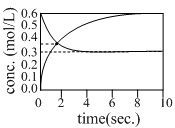The equilibrium constant K, for the above reaction is:

Solution:
QUESTION: 12

If the equilibrium reaction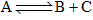is heated, it is observed that the concentration of A increases. Then,

Solution:
QUESTION: 13

Consider the reaction: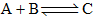The unit of the thermodynamic equilibrium constant for the reaction is:

Solution:

An equilibrium constant is defined to be equal to the ratio of the forward and backward reaction rate constants. This particular concentration quotient, , has the dimension of concentration, but the thermodynamic equilibrium constant, K, is always dimensionless.

QUESTION: 14

For the reaction H2(g)+I2(g)⇌2HI (g) at 721 K
value of equilibrium constant is 50, when molar concentration of both hydrogen and iodine is 0.5 M at equilibrium value of KpKp under the same conditions will be

Solution:
*Answer can only contain numeric values
QUESTION: 15

A 2 L vessel containing 2g of H2 gas at 27°C is connected to a 2L vessel containing 176 g of CO2 gas at 27°C. Assuming ideal behaviour of H2 and CO2, the partial pressure of H2 at equilibrium is………bar.

Solution:

H2 + CO2 - mixture of non-reacting gases
2g H2 = 1 mol
176g CO2 = 4 mol
Total no. of moles = 5
Total volume = 2+2 = 4L
Temperature = 27+273 = 300K
Total pressure, P=nRT/V=(5*0.083*300/4)= 31.125 bar
According to Dalton's law, p1 = P*(x1)
Mole fraction of H2=1/(1+4)=1/5
Therefore, p(H2)= 31.125*0.2 = 6.225 bar

QUESTION: 16

The relationship between the equilibrium constant K1 for the reaction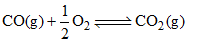And the equilibrium constant K2 for the reaction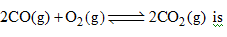Solution:
QUESTION: 17

The hydrolysis constant (Kh) of NH4Cl is 5.6×10–10. The concentration of H3O+ in a 0.1 M solution of NH4Cl at equilibrium is:

Solution:
QUESTION: 18

The equilibrium constant Kc for the following reaction at 842°C is 7.90 × 10–3. What is Kp at same temperature: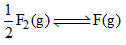Solution:
QUESTION: 19

For the equilibrium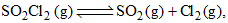what is the temperature at which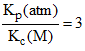Solution:
QUESTION: 20

At a certain temperature, the following reactions have the equilibrium constants as shown below: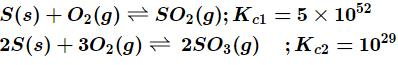What is the equilibrium constant, Kc​ for the reaction at the same temperature?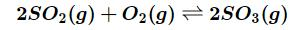Solution:

Equilibrium reactions depend upon the reaction equationsThe Kc​ for the reaction is calculated as,By the equation,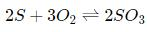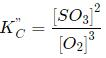By reversing the first equation and doubling the coefficient Kc

(KC,​)−1

Multiply both side of the equation  by K,

2.5×1076

QUESTION: 21

Consider the following gaseous equilibria given below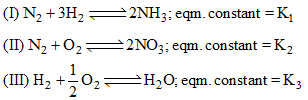The equilibrium constant for the reaction,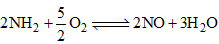in terms of K1, K2 and K3 will be

Solution:
QUESTION: 22

9.2 grams of N2O4(g) is taken in a closed on litre vessel and heated till the following equilibrium is reached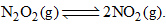At equilibrium, 50% N2O4(g) is dissociated. What is the equilibrium constant (in mol litre–1) (molecular weight of N2O4 = 92)

Solution:
QUESTION: 23

In a chemical reaction: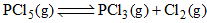xenon gas is added at constant volume. The equilibrium:

Solution:
QUESTION: 24

The equilibrium constants for the reactions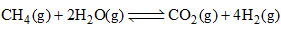and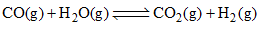are K1 and K2, respectively. The equilibrium constant for the reaction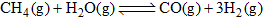is

Solution:
QUESTION: 25

Correct statement on the effect of addition of aq. HCl on the equilibrium is: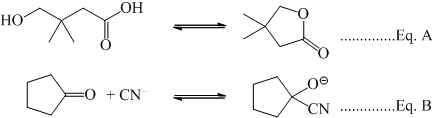Solution: